# Monogenic semi-group

(diff) ← Older revision | Latest revision (diff) | Newer revision → (diff)
A semi-group generated by one element. The monogenic semi-group generated by an elementis usually denoted by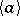(sometimes by) and consists of all powers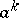with natural exponents. If all these powers are distinct, thenis isomorphic to the additive semi-group of natural numbers. Otherwiseis finite, and then the number of elements in it is called the order of the semi-group, and also the order of the element. Ifis infinite, thenis said to have infinite order. For a finite monogenic semi-groupthere is a smallest numberwith the property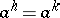, for some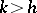;is called the index of the element(and also the index of the semi-group). In this connection, ifis the smallest number with the property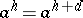, thenis called the period of(of). The pair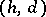is called the type of(of). For any natural numbersandthere is a monogenic semi-group of type; two finite monogenic semi-groups are isomorphic if and only if their types coincide. Ifis the type of a monogenic semi-group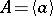, thenare distinct elements and, consequently, the order ofis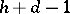; the set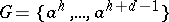is the largest subgroup and smallest ideal in; the identityof the groupis the unique idempotent in, where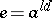for anysuch that;is a cyclic group, a generator being, for example,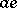. An idempotent of a monogenic semi-group is a unit (zero) in it if and only if its index (respectively, period) is equal to 1; this is equivalent to the given monogenic semi-group being a group (respectively, a nilpotent semi-group). Every sub-semi-group of the infinite monogenic semi-group is finitely generated.